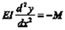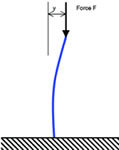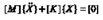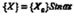## Fundamentals of Numerical Techniques for Static, Dynamic and Transient Analyses - Part 2# Fundamentals of Numerical Techniques for Static, Dynamic and Transient Analyses – Part 2

This article discusses linear buckling, transient vibration and the difference between explicit and implicit codes.

## Buckling

Most textbooks on statics or strength of materials consider the simple strut (known as the ‘Euler’ strut). The buckling load is obtained by considering the beam bending equation:This equation is also used to obtain values for lateral deflections of the beam, by integrating twice to get y in terms of moment M and distance along beam, x. As mentioned in the last article however, the solution for buckling obtained from this equation has a trigonometric form (i.e. involving Sin and Cosine functions). To obtain the buckling load, it is assumed that the buckled beam has a shape defined by the bending equation. It follows from this that the applied load F, due to its eccentricity, causes the moment M (i.e. F.y = M).

M = F.y is substituted into the bending equation, to give a second order differential equation in y, the solution of which is known to be trigonometric. The noteworthy part of this approach is that the buckled shape of the beam has been assumed beforehand. In the preceding article it was stated that, to carry out a modal frequency solution in FEA, the equation:requires an assumption that the displacement vector {X} is simple harmonic:The connection between Euler buckling and modal analysis can now be seen: the solution is trigonometric. For a modal analysis, the equations of motion have a time-based, trigonometric solution for {X}. For the Euler buckling problem, static equations are derived with an assumed trigonometric form for {X}, in terms of the spatial co-ordinates.

As for a modal analysis, there are multiple results from a buckling FE analysis, consisting of a number of buckling load factors and corresponding mode shapes. The lowest load factor is usually the only one of practical interest.

The simple linear buckling method described does not take into account any initial defects in the structure and so the results are rarely conservative: that is to say that this type of solution usually over-estimates the buckling load. In practice it may be necessary to carry out a non-linear displacement analysis, using either an explicit or implicit solution.

## Time based analyses – Implicit and Explict Solvers

Many types of non-linear analysis, like modelling slow contact between separate parts, for example, will require an analysis where the load is applied in several steps. In reality, the time over which the load is applied is not relevant but, for convenience, the steps in the analysis are applied over a specified time period of say one or ten seconds (sometimes called pseudo-time). This can be contrasted with a transient vibration analysis for example, where the real time over which the load is applied (and hence its frequency components) is fundamental to the analysis.

Both of the above problems can be carried out by finite element solution codes that use an ‘implicit’ method. The majority of mainstream and ‘traditional’ FE codes use this method. Other codes, many of which have been developed to deal with high-velocity impact problems like crashworthiness analyses, for example, use ‘explicit’ methods. The terms ‘explicit’ and ‘implicit’ refer to the numerical iteration technique used by which, having found a solution at one-time step, the solution at the next is obtained.

In an implicit solution, global equilibrium is achieved by iteration, after which local element variables are evaluated. Providing equilibrium can be achieved, there is no limit to the size of the time step that can be used. Hence, implicit schemes are termed ‘unconditionally stable’. Achieving global equilibrium at each time step involves matrix factorisation, however, which is computationally intensive. Analyses that are well-suited to implicit solution techniques are static, low-speed dynamic, or steady-state transport analyses.

By contrast, explicit solution techniques evaluate local variables directly, without the need for global equilibrium calculations. This benefit is offset by the need for calculations to be performed at small increments in order to maintain numerical stability. Significant errors develop if the time step size is too large. Hence, explicit schemes are termed ‘conditionally stable.’ Problems well suited to explicit solution are simultaneous large displacement and contact problems, rapidly changing or discontinuous loading, and rigid body motion.

Go to the next Knowledge Base article: Assessing Errors in Analysis Models

or go back to Knowledge Base article series list